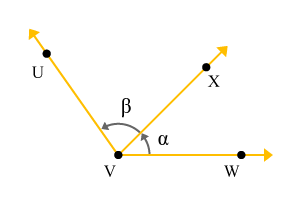Two angles formed by the three lines which have a common arm and a common vertex, are defined adjacent angles.

Two angles can be formed by the lines which are started from a point. Three lines should be required to form two angles and the three lines form two angles only when one of endpoints of three lines are connected at a point and the other endpoints of them are located at different positions on a plane.

The point at which one of endpoints of three lines are joined, is called a common vertex. The middle line becomes a common arm to the first and last lines. The first and last lines make two angles with a common arm and they are adjacent to each other. Due to this reason, the angles are called adjacent angles.

## Example

Consider three lines and join one of the endpoints of them at a point, which is called a vertex. Let us call it point $V$. one of points on the lines are located at $U$, $W$ and $X$ on a plane. Thus, three rays $\stackrel{\to }{VW}$, $\stackrel{\to }{VX}$ and $\stackrel{\to }{VU}$ are formed geometrically on a plane.

Assume, the angle between the rays $\stackrel{\to }{VW}$ and $\stackrel{\to }{VX}$ is $\alpha$. In other words $\angle XVW=\alpha$

Assume, the angle between the rays $\stackrel{\to }{VU}$ and $\stackrel{\to }{VX}$ is $\beta$. In other words $\angle UVX=\beta$

Observe the figure and the ray $\stackrel{\to }{VX}$ is a common arm which helps the other rays to make two angles but all three rays are started from a common vertex ($V$). Therefore, the two angles $\alpha$ and $\beta$ are called adjacent angles.

Latest Math Topics
Jun 26, 2023
Jun 23, 2023

###### Math Questions

The math problems with solutions to learn how to solve a problem.

Learn solutions

Practice now

###### Math Videos

The math videos tutorials with visual graphics to learn every concept.

Watch now

###### Subscribe us

Get the latest math updates from the Math Doubts by subscribing us.